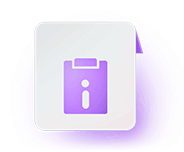• Written By Khushbu

# NCERT Solutions for Class 6 Mathematics Chapter 9 Data HandlingNCERT Solutions For Class 6 Mathematics Chapter 9 Data Handling: In this article, we bring you NCERT Solutions for Class 6 Maths Chapter 9, Data Handling. Top academic experts at Embibe prepare solutions by adopting the easiest techniques that students can understand. Therefore, students can access the solutions and practice questions to gain an edge over their preparation.

The chapter – Data Handling discusses data and ways to represent data. Moreover, the topics covered in the chapter have practice questions to help students master the concepts. In fact, there are over 1000 questions included in this chapter that students can use to practise constantly.

## NCERT Solutions for Class 6 Mathematics Chapter 9: Important Topics

Each NCERT solution in this article is provided with maximum accuracy to clear students’ doubts on Class 6 Maths Chapter 9. The NCERT Solutions constitutes important topics like Introduction to Data Handling, Bar Graph, and Pictograph. Each topic has around 250 questions curated from 20+ books.

The table below lists the important topics of Class 6 Maths Chapter 9. Furthermore, Embibe provides 10 other books for students to refer to. Also, they can enhance their understanding by referring to Embibe explainers, 3D interactive videos, and exemplars. Additionally, they can take personalised mock tests according to their level. Check NCERT Solutions for Class 6 Mathematics Chapter 9 Data Handling PDF

### NCERT Solutions for Class 6 Mathematics Chapter 9: Points to Remember

While a thorough understanding of the concepts is necessary, referring to important points is crucial when revising. Below, we have summarised a few points to remember from the chapter – Data Handling.

• Data is a collection of numbers gathered to give some information.
• Tally Mark: To get particular information from the given data quickly, the data can be arranged in a tabular form using Tally marks.
• In a Bar Graph, bars of uniform width is drawn horizontally or vertically with equal spacing between them.
• The length of each bar gives the required information.

## NCERT Solutions for Class 6 Mathematics: All Chapters

Now, students can go through the chapter-wise details along with the NCERT exercises and solutions.

## FAQs on NCERT Solutions For Class 6 Mathematics Chapter 9

Q.1: Where can I get NCERT Solutions for Class 6 Mathematics Chapter 9?

Ans: You can get NCERT Solutions for Class 6 Mathematics Chapter 9 from this page.

Q.2: How many topics are there in the chapter – Data Handling of Class 6?

Ans: There are 7 main topics covered in the chapter – Data Handling. Students can find them on this page.

Q.3: How many practice questions does Embibe provide in the NCERT Solutions for Maths Class 6 Chapter 9?

Ans: Embibe provides approximately 1000 questions to practise in the NCERT Solutions for Maths Class 6 Chapter 9.

Q.4: What is Data?

Ans: Data is a collection of numbers gathered to give some information.

Q.5: What is a pictograph?

Ans:  Pictograph represents data in the form of pictures, objects, or parts of objects.